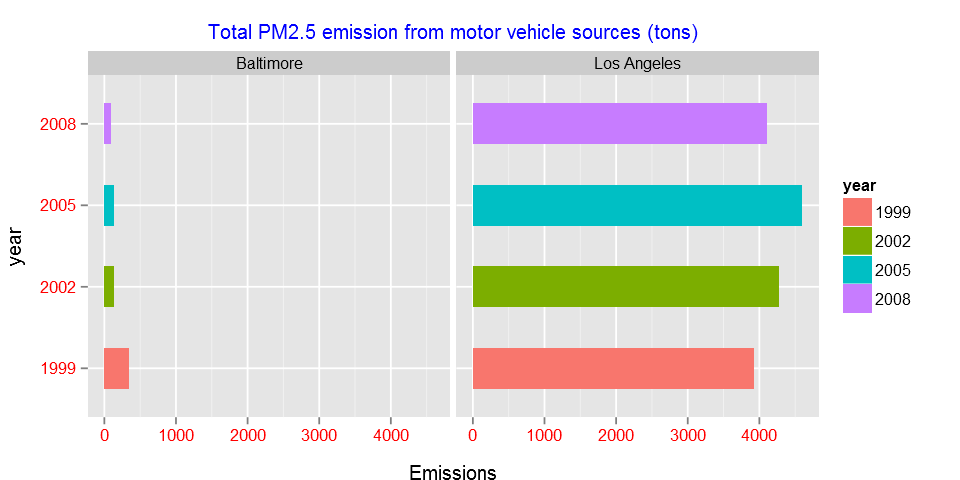# Fisseha Berhane, PhD

#### Data Scientist443-970-2353[email protected] CV Resume## Exploratory Analysis of Fine Particulate Matter¶

Fine particulate matter (PM2.5) is an ambient air pollutant for which there is strong evidence that it is harmful to human health. In the United States, the Environmental Protection Agency (EPA) is tasked with setting national ambient air quality standards for fine PM and for tracking the emissions of this pollutant into the atmosphere. Approximatly every 3 years, the EPA releases its database on emissions of PM2.5. This database is known as the National Emissions Inventory (NEI). You can read more information about the NEI at the EPA National Emissions Inventory web site.

For each year and for each type of PM source, the NEI records how many tons of PM2.5 were emitted from that source over the course of the entire year. The data that you will use for this assignment are for 1999, 2002, 2005, and 2008.

The zip file contains two files:

PM2.5 Emissions Data (summarySCC_PM25.rds): This file contains a data frame with all of the PM2.5 emissions data for 1999, 2002, 2005, and 2008. For each year, the table contains number of tons of PM2.5 emitted from a specific type of source for the entire year. Here are the first few rows.

-fips: A five-digit number (represented as a string) indicating the U.S. county

-SCC: The name of the source as indicated by a digit string (see source code classification table)

-Pollutant: A string indicating the pollutant

-Emissions: Amount of PM2.5 emitted, in tons

-year: The year of emissions recorded

Source Classification Code Table (Source_Classification_Code.rds): This table provides a mapping from the SCC digit strings in the Emissions table to the actual name of the PM2.5 source. The sources are categorized in a few different ways from more general to more specific and you may choose to explore whatever categories you think are most useful. For example, source “10100101” is known as “Ext Comb /Electric Gen /Anthracite Coal /Pulverized Coal”.

You can read each of the two files using the readRDS() function in R. For example, reading in each file can be done with the following code:

In :
setwd("C:/Fish/classes/summer_2015/Exploratory_analysis/projects/project2")


In :
str(NEI)

'data.frame':	6497651 obs. of  6 variables:
$fips : chr "09001" "09001" "09001" "09001" ...$ SCC      : chr  "10100401" "10100404" "10100501" "10200401" ...
$Pollutant: chr "PM25-PRI" "PM25-PRI" "PM25-PRI" "PM25-PRI" ...$ Emissions: num  15.714 234.178 0.128 2.036 0.388 ...
$type : chr "POINT" "POINT" "POINT" "POINT" ...$ year     : int  1999 1999 1999 1999 1999 1999 1999 1999 1999 1999 ...

In :
dim(SCC)

Out:
1. 11717
2. 15
In :
unique(NEI$type)  Out: 1. "POINT" 2. "NONPOINT" 3. "ON-ROAD" 4. "NON-ROAD" In : unique(NEI$year)

Out:
1. 1999
2. 2002
3. 2005
4. 2008
In :
str(SCC)

'data.frame':	11717 obs. of  15 variables:
$SCC : Factor w/ 11717 levels "10100101","10100102",..: 1 2 3 4 5 6 7 8 9 10 ...$ Data.Category      : Factor w/ 6 levels "Biogenic","Event",..: 6 6 6 6 6 6 6 6 6 6 ...
$Short.Name : Factor w/ 11238 levels "","2,4-D Salts and Esters Prod /Process Vents, 2,4-D Recovery: Filtration",..: 3283 3284 3293 3291 3290 3294 3295 3296 3292 3289 ...$ EI.Sector          : Factor w/ 59 levels "Agriculture - Crops & Livestock Dust",..: 18 18 18 18 18 18 18 18 18 18 ...
$Option.Group : Factor w/ 25 levels "","C/I Kerosene",..: 1 1 1 1 1 1 1 1 1 1 ...$ Option.Set         : Factor w/ 18 levels "","A","B","B1A",..: 1 1 1 1 1 1 1 1 1 1 ...
$SCC.Level.One : Factor w/ 17 levels "Brick Kilns",..: 3 3 3 3 3 3 3 3 3 3 ...$ SCC.Level.Two      : Factor w/ 146 levels "","Agricultural Chemicals Production",..: 32 32 32 32 32 32 32 32 32 32 ...
$SCC.Level.Three : Factor w/ 1061 levels "","100% Biosolids (e.g., sewage sludge, manure, mixtures of these matls)",..: 88 88 156 156 156 156 156 156 156 156 ...$ SCC.Level.Four     : Factor w/ 6084 levels "","(NH4)2 SO4 Acid Bath System and Evaporator",..: 4455 5583 4466 4458 1341 5246 5584 5983 4461 776 ...
$Map.To : num NA NA NA NA NA NA NA NA NA NA ...$ Last.Inventory.Year: int  NA NA NA NA NA NA NA NA NA NA ...
$Created_Date : Factor w/ 57 levels "","1/27/2000 0:00:00",..: 1 1 1 1 1 1 1 1 1 1 ...$ Revised_Date       : Factor w/ 44 levels "","1/27/2000 0:00:00",..: 1 1 1 1 1 1 1 1 1 1 ...
$Usage.Notes : Factor w/ 21 levels ""," ","includes bleaching towers, washer hoods, filtrate tanks, vacuum pump exhausts",..: 1 1 1 1 1 1 1 1 1 1 ...  Have total emissions from PM2.5 decreased in the United States from 1999 to 2008? Make a plot showing the total PM2.5 emission from all sources for each of the years 1999, 2002, 2005, and 2008. In : emissions<-tapply(NEI$Emissions,NEI$year,sum)  In : annual<-data.frame(emissions) annual$year<-row.names(emissions)
row.names(annual)<-NULL

In :
library(ggplot2)
library(gridExtra)

Loading required package: grid

In :
g<-ggplot(annual, aes(x=year,y=emissions))+ggtitle("Total PM2.5 emission from all sources (tons)\n in the United States")
g<-g+geom_bar(width=.5,stat="identity",colour="#CC9980",fill="#85A3E0")

g+coord_flip()+theme(axis.text=element_text(color="red",size=10))+
theme(axis.title.x=element_text(color='black',vjust=-0.9),
axis.title.y=element_text(color='black',vjust=1.5),plot.title=element_text(color="blue",size=12,vjust=1))

Out: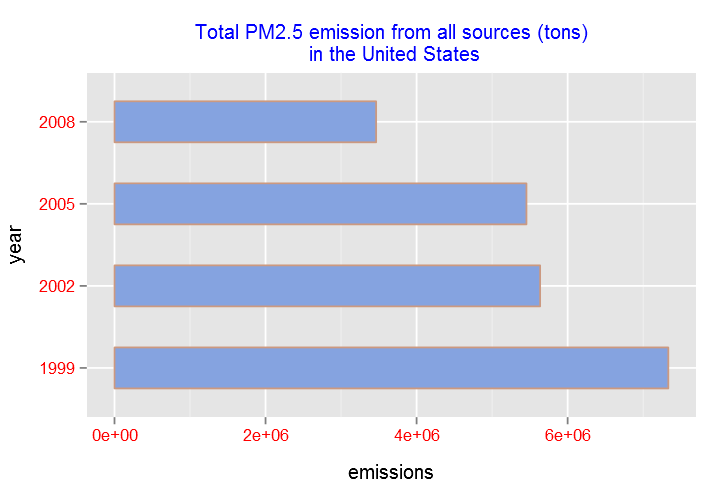We can also use the aggregate function:

In :
annual2<-aggregate(Emissions~year,NEI,sum)
annual2$year=as.factor(annual2$year)

In :
g<-ggplot(annual2, aes(x=year,y=emissions))+ggtitle("Total PM2.5 emission from all sources (tons)\n in the United States")
g<-g+geom_bar(width=.5,stat="identity",colour="#CC9980",fill="#85A3E0")

g+coord_flip()+theme(axis.text=element_text(color="red",size=10))+
theme(axis.title.x=element_text(color='black',vjust=-0.9),
axis.title.y=element_text(color='black',vjust=1.5),plot.title=element_text(color="blue",size=12,vjust=1))

Out:We can also use dplyr

In :
library(dplyr)

In :
annual3 <- tbl_df(NEI) %>%
group_by(year) %>%
summarise(Emissions = sum(as.numeric(as.character(Emissions))))
annual3$year=as.factor(annual$year)

g<-ggplot(annual3, aes(x=year,y=emissions))+ggtitle("Total PM2.5 emission from all sources (tons)\n in the United States")
g<-g+geom_bar(width=.5,stat="identity",colour="#CC9980",fill="#85A3E0")

g+coord_flip()+theme(axis.text=element_text(color="red",size=10))+
theme(axis.title.x=element_text(color='black',vjust=-0.9),
axis.title.y=element_text(color='black',vjust=1.5),plot.title=element_text(color="blue",size=12,vjust=1))

Out:Have total emissions from PM2.5 decreased in the Baltimore City, Maryland (fips == "24510") from 1999 to 2008?

In :
emissions<-tapply(NEI$Emissions[NEI$fips=="24510"],NEI$year[NEI$fips=="24510"],sum)

In :
annual<-data.frame(emissions)
annual$year<-row.names(emissions) row.names(annual)<-NULL  In : g<-ggplot(annual, aes(x=year,y=emissions))+ggtitle("Total PM2.5 emission from all sources (tons)\n in the Baltimore") g<-g+geom_bar(width=.5,stat="identity",fill="#CC9900", colour="darkgreen") g+theme(axis.text=element_text(color="red",size=10))+ theme(axis.title.x=element_text(color='black',vjust=-0.9), axis.title.y=element_text(color='black',vjust=1.5),plot.title=element_text(color="blue",size=12,vjust=1))  Out: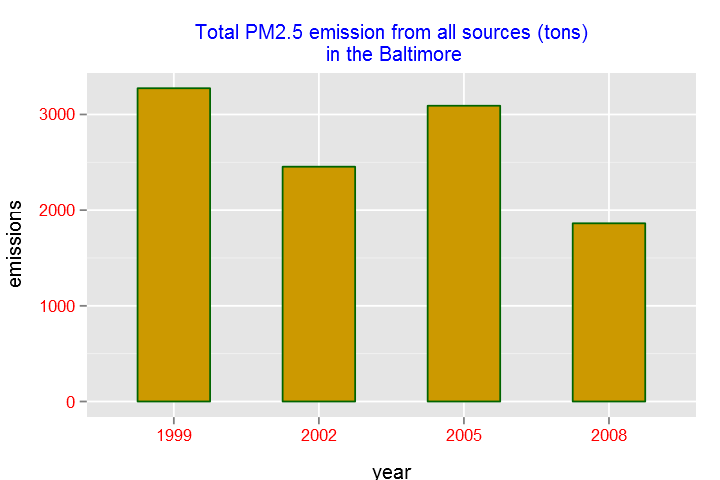Similarly, we can use aggregate: In : baltimore=NEI[NEI$fips=="24510",]
emissions2<-aggregate(Emissions~year,baltimore,sum)
emissions2$year=as.factor(emissions2$year)

In :
g<-ggplot(emissions2, aes(x=year,y=emissions))+ggtitle("Total PM2.5 emission from all sources (tons)\n in the Baltimore")
g<-g+geom_bar(width=.5,stat="identity",fill="#CC9900", colour="darkgreen")

g+theme(axis.text=element_text(color="red",size=10))+
theme(axis.title.x=element_text(color='black',vjust=-0.9),
axis.title.y=element_text(color='black',vjust=1.5),plot.title=element_text(color="blue",size=12,vjust=1))

Out:we can also use dplyr

In :
emissions3 <- tbl_df(NEI) %>%
filter(fips == "24510") %>%
group_by(year) %>%
summarise(Emissions = sum(as.numeric(as.character(Emissions))))
emissions3$year=as.factor(emissions3$year)

In :
g<-ggplot(emissions3, aes(x=year,y=emissions))+ggtitle("Total PM2.5 emission from all sources (tons)\n in the Baltimore")
g<-g+geom_bar(width=.5,stat="identity",fill="#CC9900", colour="darkgreen")

g+theme(axis.text=element_text(color="red",size=10))+
theme(axis.title.x=element_text(color='black',vjust=-0.9),
axis.title.y=element_text(color='black',vjust=1.5),plot.title=element_text(color="blue",size=12,vjust=1))

Out: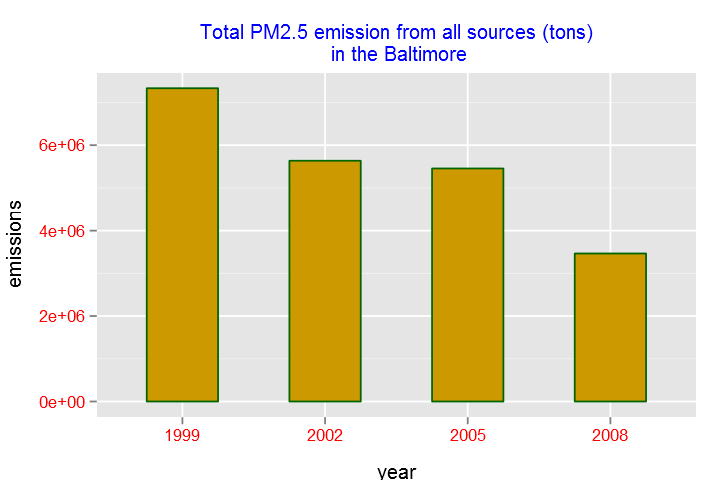Of the four types of sources indicated by the type (point, nonpoint, onroad, nonroad) variable, which of these four sources have seen decreases in emissions from 1999–2008 for Baltimore City? Which have seen increases in emissions from 1999–2008? Use the ggplot2 plotting system to make a plot answer this question.

In :
options(repr.plot.width = 8)
options(repr.plot.height = 5)

baltimore <- NEI[NEI$fips=="24510",] baltimore <- aggregate(Emissions ~ year + type, baltimore, sum) g <- ggplot(baltimore, aes(year, Emissions,color=type))+ggtitle("Total PM2.5 emission by source (in tons)\n in Baltimore") g + geom_line(size=1.25,linetype = "F1")+theme(axis.text=element_text(color="red",size=10))+ theme(axis.title.x=element_text(color='black',vjust=-0.9), axis.title.y=element_text(color='black',vjust=1.5),plot.title=element_text(color="blue",size=12,vjust=1))  Out: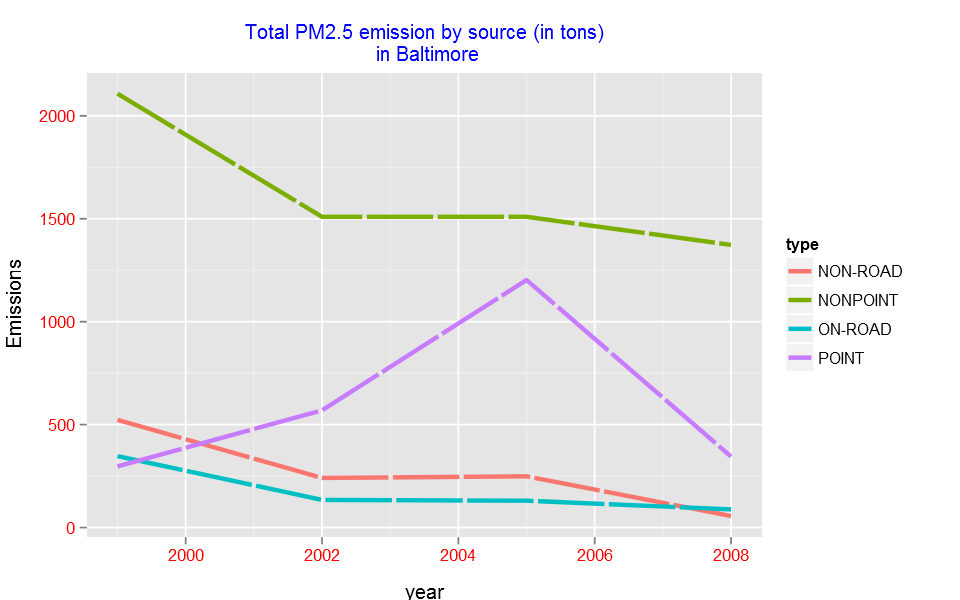Similarly using dplyr: In : baltimore <- tbl_df(NEI) %>% filter(fips == "24510") %>% group_by(year,type) %>% summarise(Emissions = sum(as.numeric(as.character(Emissions)))) g <- ggplot(baltimore, aes(year, Emissions,color=type))+ggtitle("Total PM2.5 emission by source (in tons)\n in Baltimore") g + geom_line(size=1.25,linetype = "F1")+theme(axis.text=element_text(color="red",size=10))+ theme(axis.title.x=element_text(color='black',vjust=-0.9), axis.title.y=element_text(color='black',vjust=1.5),plot.title=element_text(color="blue",size=12,vjust=1))  Out:Across the United States, how have emissions from coal combustion-related sources changed from 1999–2008? In : coal_related <- grepl("coal", SCC$Short.Name, ignore.case=TRUE)|
grepl("coal", SCC$EI.Sector,ignore.case = T) coal_related <- SCC[coal_related, ] coal_related <- NEI[NEI$SCC %in% coal_related$SCC, ] annual_coal_related <- aggregate(Emissions ~ year, coal_related, sum) annual_coal_related$year=as.factor(annual_coal_related$year)  In : g<-ggplot(annual_coal_related, aes(x=year,y=Emissions))+ggtitle("Total PM2.5 emission from coal combustion-related sources (tons)\n in the United States") g<-g+geom_bar(width=.5,stat="identity",colour="#CC9980",fill="#85A3E0") g+theme(axis.text=element_text(color="red",size=10))+ theme(axis.title.x=element_text(color='black'), axis.title.y=element_text(color='black',vjust=1.5),plot.title=element_text(color="blue",size=12,vjust=1))  Out: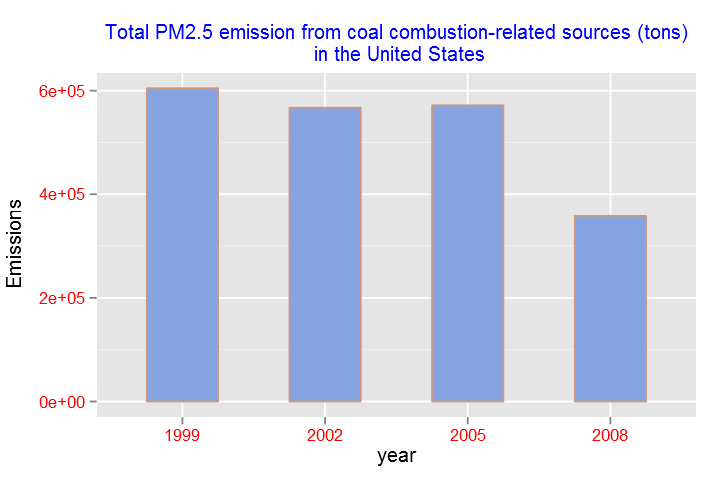or using dplyr In : coal_related <- grepl("coal", SCC$Short.Name, ignore.case=TRUE)|
grepl("coal", SCC$EI.Sector,ignore.case = T) coal_related <- SCC[coal_related, ] annual_coal_related2 <- tbl_df(NEI) %>% filter(SCC %in% coal_related$SCC) %>%
group_by(year) %>%
summarise(Emissions = sum(as.numeric(as.character(Emissions))))

annual_coal_related2$year=as.factor(annual_coal_related2$year)

g<-ggplot(annual_coal_related2, aes(x=year,y=Emissions))+ggtitle("Total PM2.5 emission from coal combustion-related sources (tons)\n in the United States")
g<-g+geom_bar(width=.5,stat="identity",colour="#CC9980",fill="#85A3E0")

g+theme(axis.text=element_text(color="red",size=10))+
theme(axis.title.x=element_text(color='black'),
axis.title.y=element_text(color='black',vjust=1.5),plot.title=element_text(color="blue",size=12,vjust=1))

Out: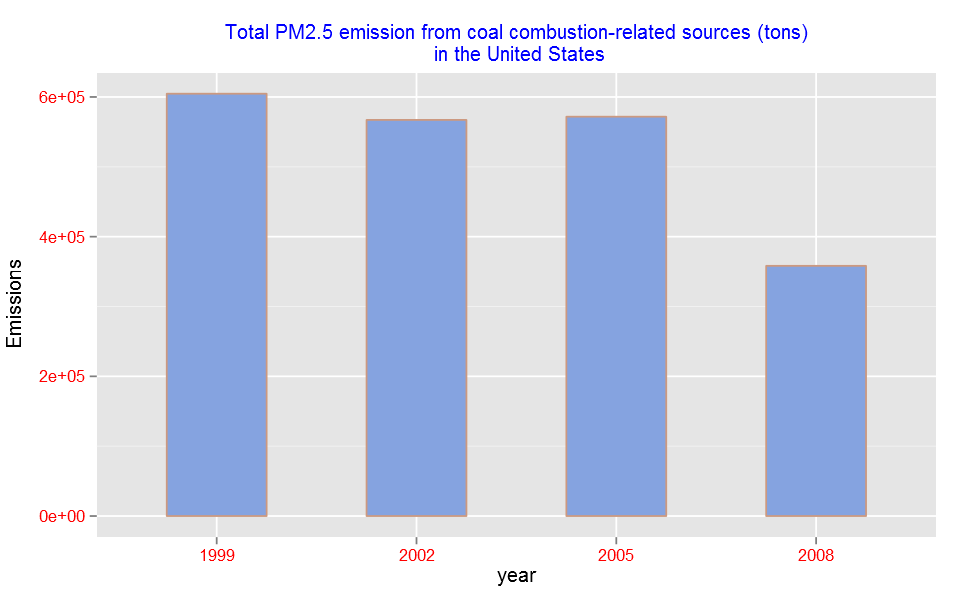How have emissions from motor vehicle sources changed from 1999–2008 in Baltimore City?

In :
balmore <- NEI[NEI$type=="ON-ROAD" & NEI$fips=="24510", ]

balmore_annual <- aggregate(Emissions ~ year, balmore, sum)
balmore_annual$year=as.factor(balmore_annual$year)

In :
g<-ggplot(balmore_annual, aes(x=year,y=Emissions))+ggtitle("Total PM2.5 emission from motor vehicle sources (tons)\n in Baltimore")
g<-g+geom_bar(width=.5,stat="identity",colour="#004C00",fill="#666699")

g+theme(axis.text=element_text(color="red",size=10))+
theme(axis.title.x=element_text(color='black'),
axis.title.y=element_text(color='black',vjust=1.5),plot.title=element_text(color="blue",size=12,vjust=1))

Out: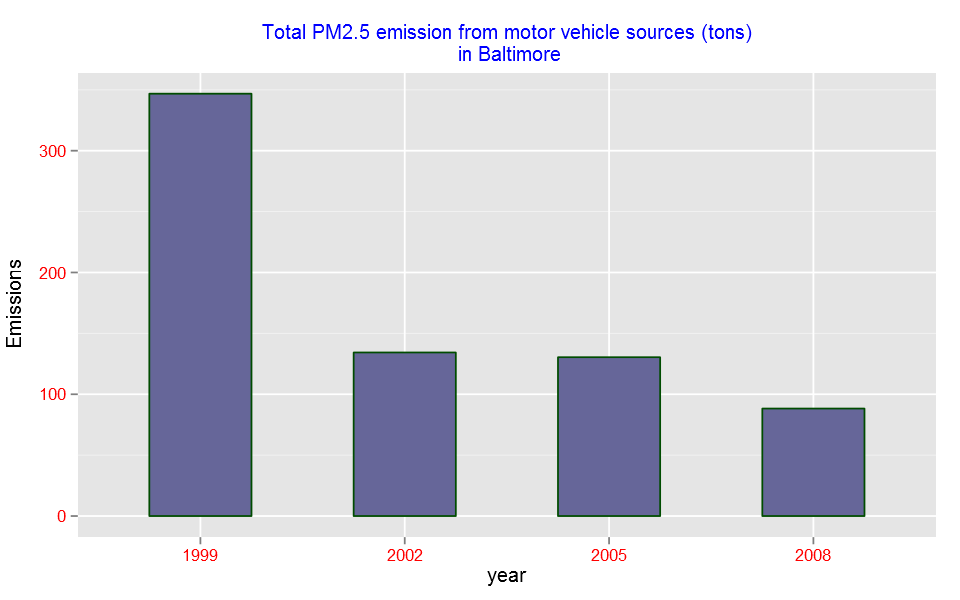Similarly with dplyr

In :
balmore_annualA <- tbl_df(NEI)%>%
group_by(year) %>%
summarise(Emissions = sum(as.numeric(as.character(Emissions))))
balmore_annualA$year=as.factor(balmore_annualA$year)

g<-ggplot(balmore_annualA, aes(x=year,y=Emissions))+ggtitle("Total PM2.5 emission from motor vehicle sources (tons)\n in Baltimore")
g<-g+geom_bar(width=.5,stat="identity",colour="#004C00",fill="#666699")

g+theme(axis.text=element_text(color="red",size=10))+
theme(axis.title.x=element_text(color='black'),
axis.title.y=element_text(color='black',vjust=1.5),plot.title=element_text(color="blue",size=12,vjust=1))

Out:Compare emissions from motor vehicle sources in Baltimore City with emissions from motor vehicle sources in Los Angeles County, California (fips == 06037). Which city has seen greater changes over time in motor vehicle emissions?

In :
bal_los <- NEI[NEI$type=="ON-ROAD" & (NEI$fips=="24510" |NEI$fips=="06037"), ] bal_los_annual <- aggregate(Emissions ~ year+fips, bal_los, sum) bal_los_annual$year=as.factor(bal_los_annual$year) bal_los_annual$fips[bal_los_annual$fips=="24510"]='Baltimore' bal_los_annual$fips[bal_los_annual\$fips=="06037"]='Los Angeles'

ggplot(bal_los_annual, aes(x=year,y=Emissions))+
ggtitle("Total PM2.5 emission from motor vehicle sources (tons)")+
geom_bar(aes(fill=year),width=.5,stat="identity")+facet_grid(.~fips)+
theme(axis.text=element_text(color="red",size=10))+coord_flip()+
theme(axis.title.x=element_text(color='black',vjust=-1),
axis.title.y=element_text(color='black',vjust=1.5),
plot.title=element_text(color="blue",size=12,vjust=1))

Out: